# Theoretical model for predicting thermoelectric properties of tin chalcogenides

Raveena Guptaab, Naveen Kumar a, Prabhjot Kaur a and Chandan Bera*a
aInstitute of Nano Science and Technology, Habitat Center, Phase-X, Mohali, Punjab-160062, India. E-mail: chandan@inst.ac.in
bCentre for Nanoscience and Nanotechnology, Panjab University, Sector-25, Chandigarh-160036, India

Received 10th June 2020 , Accepted 27th July 2020

First published on 27th July 2020

The global energy crisis demands the search for new materials for efficient thermoelectric energy conversion. Theoretical predictive modelling with experiments can expedite the global search of novel and ecoconscious thermoelectric materials. The efficiency of thermoelectric materials depends upon the thermoelectric figure of merit (ZT). In this perspective, we discuss the theoretical model to calculate thermoelectric properties. Different scattering mechanisms of electrons and phonons are calculated using a simple model for the fast prediction of thermoelectric properties. Thermoelectric properties based on the simple model have shown more than 90% agreement with the experimental values. Possibility to optimize the figure of merit by alloying, defects, nanostructuring and band convergence is also discussed for layered chalcogenides of tin. In the case of doped materials, ion-impurity scattering is found to be dominating over electron–phonon scattering and the power factor can be optimized by tuning the former scattering rate. For phonon transport, alloy scattering is found to be the most dominating among all other scattering mechanisms. Theoretically, it is found that in the temperature range between 300 K and 800 K, SnSe0.70S0.30 has the highest ZT with an efficiency of 17.20% with respect to Carnot efficiency. There could be 53.8% enhancement of the device efficiency in SnSe0.70S0.30 compared to experimentally reported SnSe0.50S0.50 in the medium temperature range (300 K to 800 K). Possible routes to achieve the best ZT in the medium temperature range are also discussed in this perspective.Raveena Gupta Raveena Gupta is working as a PhD student under Dr Chandan Bera at the Institute of Nano Science and Technology, Mohali and Panjab University, Chandigarh. Her research interest is mainly focused on the field of thermoelectricity, investigating thermoelectric properties of nanostructured chalcogenides.Naveen Kumar Naveen Kumar is currently working as a PhD student under Dr Chandan Bera at the Institute of Nano Science and Technology, Mohali. He is working on defect phase stability and thermoelectric properties of nanomaterials.Prabhjot Kaur Prabhjot Kaur obtained her PhD from the Institute of Nano Science and Technology and IISER Mohali in the field of theoretical computational physics in 2020. Her work is mainly linked with the effect of alloying and nanostructuring on thermoelectric properties.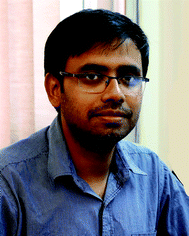Chandan Bera Dr Chandan Bera is working as a scientist in the Institute of Nano Science and Technology, Mohali. He received his MSc degree from Indian Institute of Technology, Bombay, in 2007 and PhD degree from Ecole Centrale Paris in 2010. Currently, he is working on the thermal and electron transport mechanism in alloy materials and developing a simple predictive model for thermal and electron transport in 2D and nanostructure materials for efficient design of thermoelectric devices. His group is also interested in the electron and spin transport properties of the heterostructure interface and working on the electronic and spintronic properties of the interface from the atomistic calculation based on density functional theory.

## 1 Introduction

In the present day scenario, with the increasing demand for sustainable development, the limitation of energy resources and the loss of two-thirds of used energy are becoming a major problem.1 Hence, there is a compelling need for high-performance materials that can directly and reversibly convert waste heat to electrical energy.2 Thermoelectric (TE) power generator devices convert heat energy to electrical energy using thermoelectric technology principles based on the Seebeck effect.3,4 Thermoelectric coolers, on the other hand, are based on the Peltier effect. So far the commercial market of thermoelectric devices is driven by thermoelectric coolers. Considering the high reliability of thermoelectric devices, they can also have wide applications in power generation, infrared sensors, computer chips and satellites.5 The low efficiency of these devices is a major drawback that limits their wider applications. Hence, improving thermoelectric efficiency is a key issue in this research field. Theoretical predictive modelling with experiments can accelerate the search for highly efficient TE materials. The simple theoretical model will be useful for the fast prediction of a possible route to optimize the efficiency of TE materials.

The efficiency of a thermoelectric device is given by6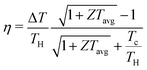(1)
where TH and TC are the hot and cold end temperatures, respectively, ΔT = THTC, Tavg = (TH + TC)/2 andis the Carnot efficiency. The efficiency of the device also depends on a dimensionless quantity known as the figure of merit (ZT) defined as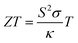(2)
where S is the Seebeck coefficient, σ is the electrical conductivity, κ is the total thermal conductivity which is the sum of lattice thermal conductivity (κl) and electronic thermal conductivity (κel) (κ = κl + κel) and T is the absolute temperature. However, an actual thermoelectric generator consists of an n-type leg and a p-type leg which are connected electrically in series and thermally in parallel.7 The figure of merit of such a device is given by8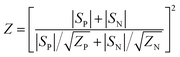(3)
where P and N stand for a p-type leg and an n-type leg respectively. The efficiency of this device is maximized when ZN = ZP and |SN| = |SP| and is then given by eqn (1).

As the fabrication of technological products has to be cost-effective, the thermoelectric materials should be available at a low price, should have high stability with respect to temperature and give sufficient output. Therefore, for enhancing ZT, proper material selection is also necessary. Almost all metals and semiconductors exhibit thermoelectricity. The high range of carrier concentration in metals causes high electrical conductivity but reduces the Seebeck coefficient. Electronic thermal conductivity of the metals also increases with increasing temperature and therefore degrades the figure of merit in metals.

Hence, semiconductor materials are the most suitable thermoelectric materials9 for getting high ZT as they have high carrier mobility and a medium range of carrier concentration.

Though many thermoelectric materials have been developed by researchers, still research is going on to approach the ideal thermoelectric materials. Thermoelectric materials are classified as chalcogenides, clathrates, skutterudites, half-Heusler (HH) compounds, silicides, oxides, Zintl phase materials, etc. based on the structure and composition of the material.10 The bar graph in Fig. 1a shows ZT for various p-type and n-type binary thermoelectric materials studied theoretically and experimentally.11–26 Among these binary compounds, tin (Sn) based materials have promising potential for commercial applications due to their low cost, high stability and average high ZT in the medium temperature range.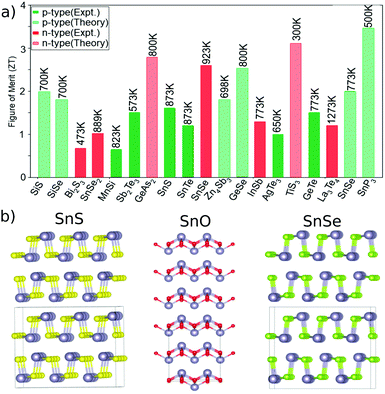Fig. 1 (a) Bar diagram showing the figure of merit of various binary p-type (green colour) and n-type (red colour) thermoelectric materials studied theoretically (striped) and experimentally (solid). The data have been taken from ref. 10–25. (b) Crystal structures of SnS, SnO and SnSe.

Theoretically studied layered SnP3 has shown the highest ZT of 3.5 at 500 K.17 Experimentally, PbTe and SnSe at temperatures above 800 K have shown ZT > 2.15 Chalcogenides have seen to be good thermoelectric materials with the potential of improving ZT by doping, alloying and nanostructuring. A recent report by Zhao et al.27 showed that Cu2Te-based compounds can be excellent thermoelectric materials if Cu deficiency is sufficiently suppressed by introducing Ag2 which increases carrier concentration and improves the ZT with a record-high value of 1.8, which is a 323% improvement over Cu2Te. Though lead-based and copper-based chalcogenides show higher efficiency, tin-based chalcogenides are drawing more attention due to their non-toxicity. Tin-based chalcogenides are being continuously explored for higher figure of merit (ZT) values by proper optimization of their structural properties.15,23,28 Out of all chalcogenides of tin, SnO, SnSe and SnS are layered and exhibit promising properties which can be explored for obtaining higher efficiency. The thermoelectric properties of these compounds can be further optimized by alloying, doping or by preparing nanostructured layers by reducing the grain size of these materials.

In this perspective, we discuss the theoretical model used for the calculation of the thermoelectric properties and various optimization methods for improving the figure of merit. The thermoelectric properties of SnS, SnSe, SnO and their alloy compounds are investigated. First-principles investigation of structural, electronic, and phonon properties of undoped and doped compounds along with their alloys is also performed using rigid band approximation and alloys of tin chalcogenides with better ZT are predicted. The theoretical model discussed here can also be applicable for other layered and non-layered compounds.

## 2 Electronic structure calculation

The thermoelectric behaviour of a material depends upon its transport properties which depend on the motion of the charge carriers. Hence, the transport coefficients are related to the electronic band structure and density of energy states of the material which can be theoretically calculated using different techniques such as density functional theory (DFT) or the tight binding model. Among these methods, DFT is one of the most popular methods to calculate electronic properties. Though many approximations are used in the DFT calculation, still it gives good agreement with the experimental electronic structure of transition metal compounds. There are many commercial and open source software based on DFT calculation. In this perspective, VASP29 (Vienna Ab initio Simulation Package) is used to do the electronic structure calculations.

The phase stable crystal structure of SnS and SnSe belongs to Pnma spacegroup while that of SnO belongs to P4/nmm spacegroup. The layered tetragonal structure of SnO with lone pair of electrons though not identical is similar to the layered orthorhombic structure of SnS and SnSe (Fig. 1b). The optimized lattice constants of SnS are a = 11.12 Å, b = 3.98 Å, c = 4.21 Å30 and that of SnSe are a = 11.49 Å, b = 4.17 Å, c = 4.29 Å30 which are in reasonable agreement with experimental reports by Chattopadhyay et al.31 and Wiedemeier et al.32 The bandgap of SnS and SnSe by GGA (Generalized Gradient Approximation) calculations is 0.62 eV and 0.30 eV respectively.30 The bandgap for both the materials improves on using the HSE06 functional30 with values 1.03 eV for SnS and 0.76 eV for SnSe, both in agreement with experimental values of 1.08 eV for SnS33 and 0.89 eV for SnSe.34

For SnO also, a semi-local Generalized Gradient Approximation – Perdew–Burke–Ernzerhof (GGA-PBE) functional35,36 with a 8 × 4 × 4 k-mesh is used. The optimized cell parameters of SnO are determined to be a = 3.77 Å, b = 3.77 Å, and c = 4.66 Å, which are in reasonable agreement with an earlier experimental report by Moreno et al.37 (a = 3.80 Å, b = 3.80 Å, and c = 4.83 Å). The GGA + PBE calculations show no bandgap for SnO, though it has an optical bandgap of 0.68 eV reported experimentally by Miller et al.38 A Hubbard-U of 6 eV is employed as an onsite correction for band structure and density of states calculation. Inclusion of U results in a bandgap of 0.34 eV which is in agreement with the theoretical value reported by Miller et al.38 (0.32 eV).

## 3 Transport model

The thermoelectric transport coefficients are calculated in the framework of the Boltzmann transport equation which gives the position and velocity of particles as its solution. The equation balances the various processes in the steady state and extracts out macroscopic physical properties of carriers in metals or semiconductors.39 In steady state, the Boltzmann equation is given by40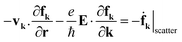(4)
where vk is the velocity of the particle in the kth state and fk is the distribution function which measures the number of carriers in state k in the neighbourhood of r. The first term on the left represents the change in distribution function due to the diffusion of carriers because of concentration or temperature gradient. The second term represents the change in distribution function due to external field E.40 The right hand side of the equation is due to various scattering processes which affect the motion of the electrons.

The scattering term of eqn (4) consists of non-linear integrals which are difficult to solve analytically; hence, numerical methods like Monte Carlo and finite element method and approximation methods like variational method and relaxation time approximation method are used to solve the transport distribution function. Some of the methods used for solving the Boltzmann transport equation are discussed briefly below.

### 3.1 Monte Carlo method

In the Monte Carlo method, the numerical solution of the Boltzmann transport equation is done based on a stochastic approach which gives the solution of the equation using band structure and scattering mechanisms. The scattering mechanism is extracted using Fermi's golden rule which gives the first order transition probability for scattering from state k to state k′ as41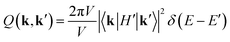(5)
where H′ is the collision Hamiltonian and E and E′ are the initial and final energies respectively. Once the scattering probability is evaluated, the scattering rate is calculated for a given process.

This method is widely used for studying the thermoelectric properties of materials. Jeng et al.42 presented a Monte Carlo simulation scheme to study the thermoelectric properties of nanocomposites with special attention paid to the implementation of periodic boundary conditions in Monte Carlo simulation. They applied the scheme to study the thermal conductivity of silicon germanium (Si-Ge) nanocomposites, which are of great interest for high efficiency thermoelectric material development. Gelmont et al.39 presented the results of an ensemble Monte Carlo simulation of the electron transport in gallium nitride (GaN) and showed that intervalley electron transfer plays a dominant role in GaN in high electric fields leading to a strongly inverted electron distribution and to a large negative differential conductance. Bera et al.43 also performed Monte Carlo simulation of thermal conductivity of Si nanowires to investigate the effect of phonon confinement on thermal transport.

### 3.2 Variational method

In this method, the approximate solution of the Boltzmann transport equation is found by applying the variational theorem. Physical interpretation of the variational theorem is that the variational function is directly proportional to the rate of entropy production by collisions or scatterings.44 Hence, the variational theorem corresponds to steady state thermodynamics in the case of non-equilibrium statistical mechanics.

Considering that the electron is under the effect of just the electric field and that the change in distribution function (f) from equilibrium distribution function (f0) is very small, that is ff0, the rate of change of distribution function is given as40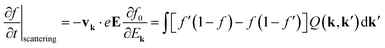(6)
where Q(k,k′) is the probability of transition from a point k to point k′ and Ek is the energy in state k. In this method, the Taylor series expansion of the distribution function is truncated after a few terms and is given by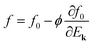(7)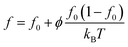(8)
where ϕ is a new function which is considered to be the solution of the Boltzmann equation which is now represented as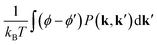(9)
where P(k,k′) is the equilibrium transition probability rate. This equation can also be written as
 X = Lϕ (10)
where X is a function of k and is electric field dependent and L is the scattering operator which transforms ϕ to another function of k.

The transport coefficients obtained in this way are always less than their exact values as the results may not converge if the power series is terminated after a small number of terms.41 The inclusion of a large number of terms makes the computation very laborious. If expansion functions other than a simple power series are considered, it may give a rapid convergence.

Very few studies are there based on the variational method due to its limitations. A report by Casian et al.45 presented a systematic theoretical analysis of electronic states and thermoelectric transport in PbTe/Pb1−xEuxTe quantum well structures by employing a more realistic well model than has been used up to now by using the variational method. The electrical conductivity, thermopower (Seebeck coefficient) and thermoelectric power factor as functions of the well width were studied for quantum well (QW) structures with (100) and (111) crystallographic orientations and different carrier densities and it was found that the power factor is greater in (100) QWs, but the more realistic the well model is, the lower is the power factor.

### 3.3 Iterative solution method

The iterative technique to solve the Boltzmann equation was first reported by Rode46 in 1970 by applying the idea of the Ritz iteration method47 used for the solution of linear algebraic equations. This method is mainly used for the case where polar optical phonon scattering is dominant. It makes the numerical calculation and the nanoscale study of heat transfer possible.

In this method the Boltzmann equation is reduced to the difference equation for the perturbation term and solutions are obtained by numerical iteration. The perturbation in the distribution function is determined by performing calculations for a number of energy points within the energy range of interest and at a particular iteration it is evaluated from the scattering-out term. The transport coefficients are hence evaluated using the perturbation. The contribution of the scattering-in term is not significant. A few iteration steps are usually required for a convergent result.

Rode utilized this method to calculate the drift mobility in several II–VI and III–V compounds.48,49 The thermoelectric powers of some III–V semiconductors were also calculated by him using this method.

### 3.4 Relaxation time approximation

Relaxation time approximation (RTA) is the most used technique in the theoretical calculation of the TE properties. In this method, the concept of relaxation time τk is used to understand the transport of electrons and holes in semiconductors. The relaxation time represents the time taken by the disturbed distribution function (f) to relax back to its equilibrium state (f0). A more detailed discussion of the RTA method can be found in ref. 41. The Boltzmann equation under this approximation is represented as50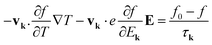(11)

The motion of an electron is unhampered in a perfect crystal in which the wave function of the electron is given by stationary Bloch functions. The application of an external field in such crystals would uniformly accelerate the electron causing a linear increase of the drift velocity with time in the direction of the field.51 Such linear increase in drift velocity with time is not observed in real crystals. The average drift velocity of the electron reaches a limiting value which at low field is proportional to the magnitude of the applied field. The limit is set by the interaction of the electron with the imperfection of the crystal through the process referred to as the scattering or collision process.

The relaxation time approximation method is widely used for evaluating the thermoelectric properties of materials.52,53 Recently, Gupta et al.30 have shown that relaxation time approximation works very well for the calculation of thermoelectric properties of SnS, SnSe and SnSe1−xSx which are in good agreement with the experimental reports.54,55

## 4 Electrical transport

The electrical transport properties are calculated using the Boltzmann transport equation within the relaxation time approximation. The Boltzmann transport equation is solved as implemented in BoltzTraP2.56 Relaxation times are calculated based on electron–phonon scattering (τe–ph), ionized-impurity scattering (τii) and alloy scattering (τal) when applicable.

In the relaxation time approximation, the generalized transport coefficients are calculated from the transport distribution function given by56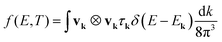(12)

In the above equation, vk is the component of the group velocity of each carrier in the transport direction, Ek is the energy of that electronic state and τk is its total relaxation time which is calculated using Matthiessen's rule, i.e.,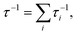where i runs over all scattering mechanisms. The moments of generalized transport coefficients are then given by56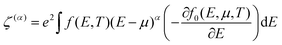(13)

The transport coefficients are obtained by applying boundary conditions. Identifying the conditions of zero temperature gradient and zero electric current, the transport coefficients are expressed as56

 σ = ζ(0) (14)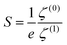(15)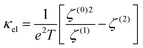(16)

Variation of the Seebeck coefficient (S) with carrier concentration (n) for layered chalcogenides (SnO, SnS and SnSe) is shown in Fig. 2. SnO shows the highest Seebeck coefficient compared to SnSe and SnS for both p-type and n-type compounds. For both p-type and n-type cases, the Seebeck coefficient decreases with an increase in carrier concentration as Sn−2/3. The p-type compounds have a Seebeck coefficient of lower magnitude compared to the n-type compounds as seen from the figure. The experimental values of the Seebeck coefficient38,54,55 are plotted in Fig. 2 for SnS, SnSe and SnO and correspond to 8.4 × 1016 cm−3, 1.5 × 1017 cm−3 and 5 × 1017 cm−3 of carrier concentration respectively.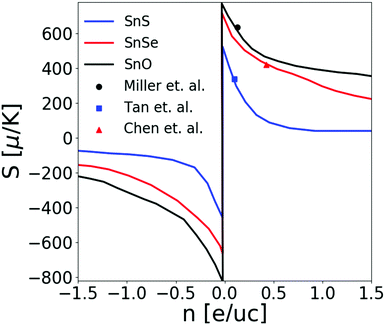Fig. 2 Variation of the Seebeck coefficient with carrier concentration (measured in electrons per unit cell [e uc−1]) for SnS, SnSe and SnO at 300 K along with experimental values38,54,55 (square – SnS, circle – SnO, triangle – SnSe).

### 4.1 Electron scattering mechanisms

The electron continues to be in a stationary state until it comes close to an imperfection. Once it reaches close enough, the electron interacts with the imperfection and acquires a new wave function characterized by a new wave vector and a different value of energy. In a lattice crystal, there are different types of scattering mechanisms for different imperfections giving rise to various scattering mechanisms like intervalley, intravalley, carrier–carrier and defect scattering as listed in Fig. 3. The importance of each kind of scattering varies not only from material to material but also depending on temperature and carrier concentration. Graziosi et al. reported the importance of the scattering physics for the power factor of half-Heuslers by extracting charge transport properties.57 Some of the electron scattering mechanisms are discussed below.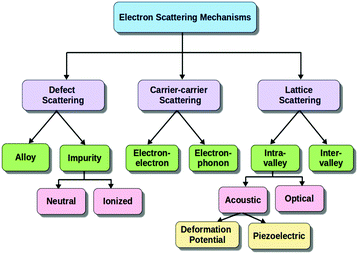Fig. 3 Electron scattering mechanism adopted from ref. 41.
4.1.1 Electron–electron scattering. The effect of electron–electron scattering is more in degenerate semiconductors and can be neglected in non-degenerate semiconductors. The effect of this scattering process due to Coulomb forces was considered by Appel et al.58 in the framework of Kohler's variation principle. By considering the conduction electrons as a Fermi–Dirac gas of non-interacting free quasi-particles, each with charge e and mass m*, they took this scattering into account as a small perturbation to evaluate transport phenomena in non-polar semiconductors.
4.1.2 Piezoelectric scattering. This scattering plays an important role and dominates deformation potential scattering at low temperatures in crystals having the piezoelectric effect. This scattering was first discussed by Meijer and Polder,59 who estimated the magnitude of the relaxation time for piezoelectric scattering in crystals with zinc blende symmetry. The piezoelectric mobility of electrons (or of holes) was also calculated by Zook60 for the three classes of known piezoelectric semiconductors, assuming energy surfaces which are ellipsoids of revolution with principal axes along the crystal axes. The piezoelectric scattering time is directly proportional to the square root of energy (E),
 τpiezo ∝ E1/2 (17)
and the mobility is dependent on the square root of temperature (T),
 μ ∝ T1/2 (18)
4.1.3 Electron–phonon scattering. Electron scattering by lattice vibration, i.e. by the phonon eigenstates, was described by Bardeen and Shockley.61 Phonons disturb the lattice, which moves the atoms from their original position in the lattice. An electron is affected by this position changing and may be liable to be deflected or scattered and hence the electron–phonon scattering rate is given by30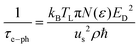(19)
where N(ε) is the electronic density of states, kB is the Boltzmann constant, TL is the lattice temperature, ρ is the density of the medium, us is the speed of sound and ED is the band deformation potential which is defined as the change in the energy of an electronic level per unit of applied strain and is given by61,62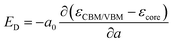(20)
where a is the lattice constant, a0 its equilibrium value, ∂(εCBM/VBMεcore) is the energy difference between the conduction band minima (CBM) or the valence band maxima (VBM) and a core level.

Electron–phonon relaxation time variation with energy (EEF) where EF is the Fermi energy is shown in Fig. 4a. As the relaxation time is inversely proportional to the density of states of the system, the electron–phonon relaxation time is the lowest for SnSe and the highest for SnO. The e–ph relaxation time is calculated with a fixed phonon velocity. For SnO thermalization of electrons is much faster compared to SnS or SnSe. This scattering mechanism was used to calculate the electrical conductivity of SnO, SnS and SnSe (Fig. 4b). The electrical conductivity values of SnO lie in between those of SnS and SnSe making it a potential oxide thermoelectric and increase with an increase in carrier concentration. All p-type compounds show electrical conductivity of higher magnitude than n-type compounds as the scattering rate for p-type compounds is less compared to n-type compounds. For SnSe, it has electrical conductivity three times higher than that of SnS and SnO due to higher concentration (∼1018 cm−3) of intrinsic charge carriers. It is a highly degenerate semiconductor and hence it shows quasi metallic features.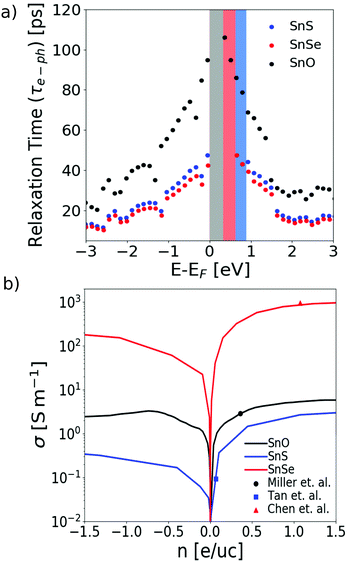Fig. 4 (a) Variation of electron–phonon relaxation time with energy (E − EF). (b) Variation of electrical conductivity with carrier concentration for pure SnO, SnSe and SnS at 300 K along with experimental values38,54,55 (square – SnS, circle – SnO, triangle – SnSe).
4.1.4 Ionized-impurity scattering. The ionized-impurity scattering rate comes into play when an impurity atom is added to an existing pure compound and it has a T3/2 partial mobility dependence,63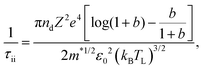(21)
with b defined as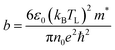(22)

In the above equation, nd is the defect concentration, Z is the atomic number of the impurity element, m* is the effective mass of the carriers and n0 is the carrier concentration.

The ion-impurity scattering dominates electron–phonon scattering in all temperature ranges as shown in Fig. 5a for SnO. Gupta et al. had shown a hundred times increase in the electrical conductivity value for SnS and a five times increase for SnSe on silver doping. The n-type dopant iodine reduced the electrical conductivity of SnSe due to the pinning of Fermi energy inside the bandgap, making it a non-degenerate semiconductor. Silver (Ag) and indium (In), which are potential p-type dopants as their impurity states stabilize the Fermi level and enhance charge collection,38 along with gallium (Ga) are used to enhance the electrical conductivity of SnO. Maximum enhancement of the electrical conductivity (from 11.5 S m−1 to 55 S m−1) for p-type SnO is observed for Ag doping (Fig. 5b). Carrier concentration after doping plays an important role in the electrical conductivity of tin chalcogenides and enhances their properties.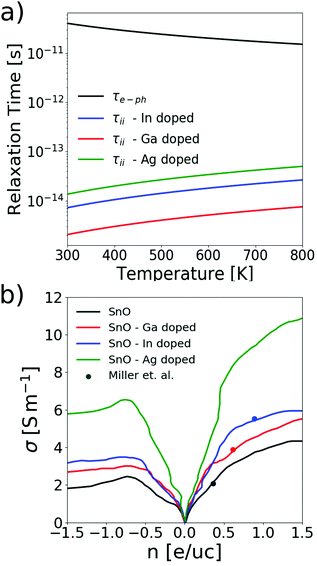Fig. 5 (a) Variation of electron–phonon relaxation time and ion-impurity relaxation time with temperature. (b) Variation of electrical conductivity with carrier concentration for pure and doped SnO at 300 K along with experimental values38 (red circle – Ga doped SnO, black circle – pure SnO, blue circle – In doped SnO).
4.1.5 Alloy scattering. In the case of alloys, mobility is affected not only by impurities but also by disorder arising from atomic positions that break the lattice periodicity. The scattering rate for alloy scattering is given as64,65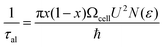(23)
where Ωcell is the unit cell volume, ħ is the reduced Planck constant and U is the alloy potential parameter whose value is considered to be 0.15 × 105 similar to that considered for the SnSe1−xSx alloy.30

Alloy scattering dominates electron–phonon scattering; however it is lower compared to impurity scattering for doped materials (Fig. 6a). The electrical properties for the SnSe1−xSx alloy showed a similar behaviour as SnS and SnSe.30 The alloy SnSe0.70S0.30 shows an increase in electrical conductivity from 0.9 S m−1 at 300 K to 100 S m−1 at 800 K upon increasing temperature. Though the electrical conductivity is increased for SnSe1−xSx compared to SnS, the values are inferior compared to that for SnSe at 300 K.30 The electrical properties for the SnO0.70S0.30 alloy and SnO0.70Se0.30 are shown in Fig. 6b. Similarly, the alloys of SnO also show an increase in electrical conductivity with temperature. We found a higher value of electrical conductivity of 30 S m−1 at 700 K for SnO0.70Se0.30 compared to 15 S m−1 for pure SnO and 20 S m−1 for the SnO0.70S0.30 alloy.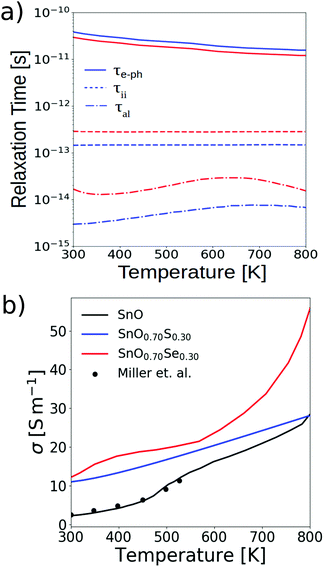Fig. 6 (a) Variation of electron–phonon relaxation time, ion-impurity relaxation time and alloy relaxation time with temperature for SnO0.70S0.30 (blue colour) and SnO0.70Se0.30 (red colour) with indium doping. (b) Variation of electrical conductivity with temperature for pure SnO, SnO0.70S0.30 and SnO0.70Se0.30 along with experimental values38 for pure SnO.
4.1.6 Intervalley scattering. For the indirect band crystals, besides the above mentioned scattering, intervalley scattering which is the transition of electrons between states in different conduction band valleys is also important. This scattering can be formally treated in the same way as intravalley scattering by optical phonons.66 This scattering is included in a report by Bera et al.52 for the Si-Ge case by assuming that various indirect equivalent minima are isotropic and parabolic and is given as52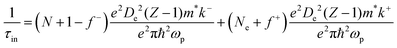(24)
where N is the total number of carriers, Z is the number of isotropic valleys at each band, De is the intervalley potential, f± is the distribution function evaluated at energy δ′ = δ ± ħωp and ħωp is the phonon energy. The intervalley scattering rate is proportional to k′ and has a T−3/2 mobility dependence.

The electronic transport properties and relaxation time for different scattering mechanisms can be also calculated using first-principles methods.67 Samsonidze et al.68 first principles calculations of the electron–phonon coupling demonstrated that the energy dependence of the electron relaxation time varies significantly with chemical composition and carrier concentration. Wang et al.69 combined the Boltzmann transport equation with an ab initio approach to compute the thermoelectric coefficients of semiconductors like silicon by taking electron–phonon, ionized impurity, and electron–plasmon scattering mechanisms into account. In another report70 the electrical conductivity accumulation with respect to electron mean free paths is compared to phonon thermal conductivity accumulation by using first-principles simulation and the thermoelectric properties of the bulk and nanostructured silicon are predicted. PERTURBO71 an open source software which mainly computes electron–phonon (e–ph) interactions and phonon limited transport properties in the framework of the Boltzmann transport equation (BTE) has recently been released to calculate the thermoelectric properties of materials using first-principles calculations and Wannier functions.

## 5 Phonon transport

The performance of thermoelectric materials not only depends on the electrical transport properties but also strongly depends on the thermal conductivity of the material. Hence, understanding the transport of phonons is very important to design efficient thermoelectric devices. Under non-equilibrium conditions, the distribution of phonons deviates from their distribution in equilibrium conditions. The transport of phonons is computed using the Boltzmann transport formalism. The force constants are extracted using a real-space approach as implemented in the PHONOPY package62 by using the finite difference method (frozen phonon approach) or the linear response method (DFPT (density functional perturbation theory) approach). Thermal conductivity calculations are done once the phonon modes are obtained and force constants are calculated.

Within the relaxation time approximation, the lattice thermal conductivity is given by72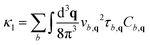(25)
where the sum is over all phonon bands b, the integral is over all the Brillouin zones, vb,q is the group velocity of a given phonon mode along the chosen direction, τb,q is the mode relaxation time and Cb,q is the mode heat capacity, dependent on the mode frequency ωb,q and temperature. vb,q and Cb,q are directly calculated from the phonon band structure.

The relaxation time is calculated for different scattering mechanisms like Umklapp scattering and Normal scattering processes. Normal scattering becomes important in materials having a layered structure30 where anharmonic scattering overestimates the thermal conductivity.73 Alloy scattering and boundary scattering of phonons are also included in the calculations.

### 5.1 Phonon scattering mechanisms

Phonons when they travel through the material can scatter due to other phonons, impurities, electrons and boundary of the material. The various scattering mechanisms are discussed in the following sections.
5.1.1 Umklapp (U) scattering. The U-process is a phonon–phonon scattering process which is the most dominant process at high frequencies and high temperatures for crystals having less defects. The schematic of this process is shown in Fig. 7a where two large incoming phonons with wavevectors k1 and k2 result in an outgoing phonon with wave-vector k3′ pointing outside the first Brillouin zone.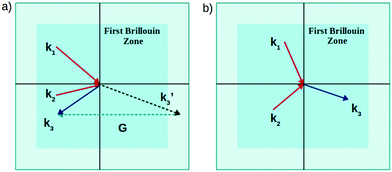Fig. 7 Schematic diagram of the (a) U-process and (b) N-process.

Hence, the net phonon momentum is not conserved in this process. The wave-vector is then mathematically transformed (k3) to a point inside the first Brillouin zone. This transformation allows for scattering processes to physically occur. It is treated in the quasi-harmonic approximation which is based on the assumption that the harmonic approximation holds for every value of the lattice constant, which is viewed as an adjustable parameter. The scattering under this approximation is given by7,72,73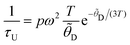(26)
where p is an adjustable parameter given by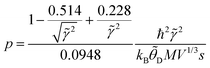(27)
and V is the unit cell volume, s is the speed of sound, M is the average atomic mass and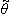D is the Debye temperature defined by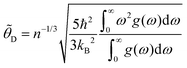(28)

In the above equation, n is the number of atoms per unit cell and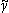2; is the mode averaged-squared Grüneisen parameter given by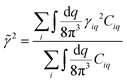(29)

The volume derivatives necessary to obtain the Grüneisen parameter are calculated for a specific volume range using the quasi-harmonic approximation. From the calculation of atomistic contribution of the Grüneisen parameter of SnO (Fig. 8a), SnS (Fig. 8b), and SnSe (Fig. 8c) by each of their constituent atoms, it is found that in SnO, the contribution of O atoms is higher in lower energy phonon modes while Sn contributes more in higher energy modes. In SnS, both atoms, Sn and S, contribute equally and the same is also seen for SnSe.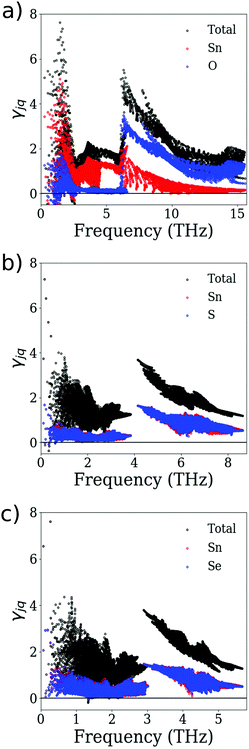Fig. 8 Atomistic contribution of the Grüneisen parameter in (a) SnO, (b) SnS and (c) SnSe.

The Umklapp scattering model based on Grüneisen parameter calculations was also reported by Toher et al.74 and Toberer et al.75 for different structures. Miller and Toberer et al.76 used empirical fitting to calculate this parameter.

Inclusion of this scattering mechanism gives lattice thermal conductivity values near to experimental cases73 except for the case of layered Sn-chalcogenides where only inclusion of this scattering overestimates the thermal conductivity as reported by Gupta et al.30

5.1.2 Normal (N) scattering. The normal scattering plays an important role for layered chalcogenides along with the U-process. In the N-process, the net phonon momentum (G) is conserved. The schematic is given in Fig. 7b, where the sum of incoming phonon wave-vectors remains inside the first Brillouin zone.

The scattering rate is calculated as77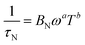(30)
where BN is the normal phonon scattering rate coefficient, which for different a and b can be written as77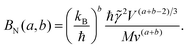(31)

The parameters a and b are adjustable and depend on the material's structure. The values are chosen to be a = 0.5 and b = 1.5 for thermal conductivity calculations of SnS, SnSe and SnO.

5.1.3 Defect scattering. Scattering of phonons by point defects in crystals has been treated theoretically using perturbation theory and by self-consistent methods using Green function techniques by Klemens et al.78 These self-consistent methods are needed when the incident phonons are near or above an intrinsic resonance frequency of the defect. At sufficiently low frequencies, it is expected that perturbation theory will give good results if the unperturbed Hamiltonian of the system is chosen to minimize the perturbation. A defect or vacancy (V) gives rise to a mass difference equal to the mass of the missing atom, Mv. The breaking of bonds contributes in a way corresponding to a mass difference of 2M. This at low frequencies will cause a relaxation time given by79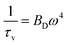(32)
where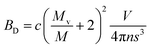(33)

In the above equation V/n is the volume per atom and s is the speed of sound.

5.1.4 Alloy scattering. Alloy scattering is incorporated into phonon transport by taking into account the mass disorder in the alloy.7 This scattering rate is calculated as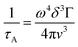(34)
where v is the phonon velocity in the alloy, Γ is the scattering cross-section Γ = x(1 − x)(ΔM/M),2 ΔM = MAMB and M = xMA + (1 − x)MB, MA and MB being the mass of alloy components A and B respectively. δ3 is the atomic volume of the alloy estimated as a weighted average,
 δ3 = xVA + (1 − x)VB (35)
where VA and VB are the atomic volumes of components A and B.

For an alloy, the total anharmonic and normal scattering rate is calculated as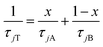(36)
where A and B are the two components of the alloy and j stands for anharmonic or normal scattering.

5.1.5 Boundary scattering. This scattering plays an important role for low-dimensional nanostructures and dominates over other scattering mechanisms. The boundary scattering is given by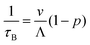(37)
where v is the phonon velocity, Λ is the characteristic length of the system and p represents the fraction of specularly scattered phonons. The parameter p can be computed using40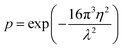(38)

In the above equation, η is the root-mean-square roughness of the surface and λ is the wavelength.

We use a diffusive model80 in Sn-based chalcogenide systems taking p = 0 and hence, the scattering rate reduces to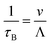(39)

The phonon modes in SnO have the maximum scattering rate 1.54 × 1012 s−1 compared to SnS and SnSe which leads to a maximum mean free path of 3.19 μm at 300 K. The relaxation time and the mean free path of the phonons decrease with temperature leading to a decrease of lattice thermal conductivity with temperature. Variation of thermal conductivity with characteristic length Λ for SnO, SnS and SnSe is shown in Fig. 9. SnO also shows a similar behaviour like SnS and SnSe30 with values varying from 1.78 to 1.60 as the characteristic length of the particle is reduced to 20 nm. Considering the diffusive type boundary scattering in these materials, the phonon mean free path is already very low, hence the sample sizes in the nanoscale have very little impact on thermal conductivity.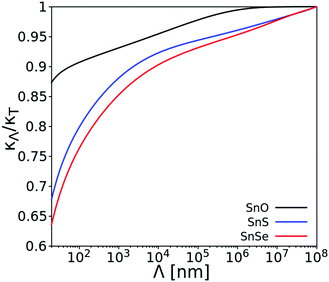Fig. 9 Variation of κΛ/κT with characteristic length of the material – SnS, SnO and SnSe.

Fig. 10a shows the phonon band structure of SnO. Tin based chalcogenides have been reported to have thermal conductivity less than 5 W m−1 K−1. Experimentally, SnTe28 has been seen to show the highest lattice thermal conductivity of 3.7 W m−1 K−1 compared to its counterparts at room temperature. SnSe has been reported to have an ultralow lattice thermal conductivity of 0.4 W m−1 K−1 at 300 K as shown by Zhao et al.15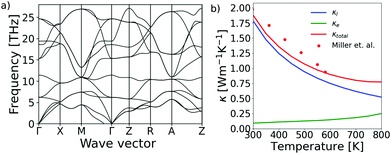Fig. 10 (a) Phonon band structure of SnO. (b) Variation of lattice thermal conductivity, electronic thermal conductivity and total thermal conductivity with temperature of pure SnO.

An experimental report55 showed SnSe to have a thermal conductivity of ∼1.27 W m−1 K−1 at 300 K. When only umklapp scattering is considered by the designed model, a very high value of 7.3 W m−1 K−1 is obtained. A similar observation is made in the case of SnS too.30 SnO has a thermal conductivity of 1.97 W m−1 K−1 as reported by Miller et al.

From our model, only U-processes overestimate the thermal conductivity values of layered chalcogenides. Therefore, by including the N-process as shown in Fig. 10b, the lattice thermal conductivity comes out to be well in agreement with the experimental value.

Fig. 11 shows the projected densities of states (PDOS) for each element in each structure SnS, SnSe and SnO. Starting from the low frequencies, the density of states is dominated by the massive Sn atom for all the three compounds SnS, SnSe and SnO. The contribution from the chalcogen atoms dominates in the higher frequency region. The Se atom has almost the same contribution as the Sn atom in SnSe while S and O atoms contribute less.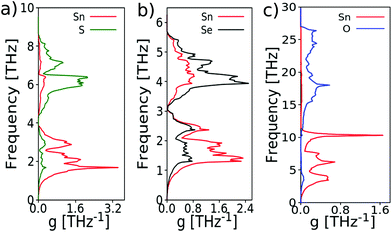Fig. 11 Projected density of states, colored by respective elements, in each of the three structures (a) SnS (b) SnSe and (c) SnO.

The lattice thermal conductivity can also be obtained by performing full ab initio calculations by using almaBTE83 or ShengBTE84 which are based on three-phonon processes using third order force constants. In a recent report,85 the lattice thermal conductivity of highly doped silicon was calculated considering the electron–phonon and defect scattering mechanisms both individually and together using first-principles. These ab initio techniques though accurate are very time consuming and based on a tedious calculation procedure. Fig. 12 shows the thermal conductivity obtained by different models compared with the experimental value. From these calculations, it is observed that inclusion of normal scattering processes gives results well in agreement with experimental values. It also shows that a simple model gives results comparable to the full ab initio based calculations in a much more faster way.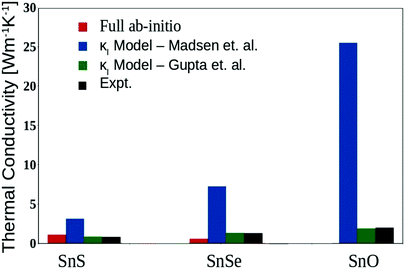Fig. 12 Bar plot showing a comparison of the thermal conductivity of SnS, SnSe, and SnO using different models30,73 and experimental values.20,38,81,82

## 6 Optimization of figure of Merit

The figure of merit (ZT) can be increased by increasing electrical conductivity and the Seebeck coefficient and decreasing thermal conductivity. It is not easy to improve ZT as all these coefficients are coupled with each other and are strongly dependent on the material's crystal structure, electronic structure and carrier concentration. For a material to show good thermoelectric performance, not only a high figure of merit over a wide operating temperature range is required but also mechanical, metallurgical and thermal characteristics are necessary in practical thermoelectric generators.

There are various ways in which the thermoelectric properties of thermoelectric materials can be improved to obtain higher efficiency of thermoelectric devices. Some of the ways of enhancing the ZT of Sn-based chalcogenides are discussed in the following sections.

### 6.1 Intrinsic and extrinsic defects

The high intrinsic resistivity of all undoped Sn chalcogenides indicates that doping or extrinsic defects must be introduced. Defects play a very important role in mediating self-diffusion and the diffusion of substitutional impurities in semiconductors. They also optimize the carrier concentration. In a heavily doped semiconductor, the charge concentration is controlled by the formation of charge defects and there exists a well-defined theoretical approach for this study.86,87 The defect engineering involves the study of both extrinsic defects and intrinsic defects (vacancies, interstitials and antisite defects). The amount of carriers that can be generated in a semiconductor depends on its detailed defect thermochemistry and there should not be any compensating or killer intrinsic defects. The killer defects produce the oppositely charged carriers, thereby pinning the chemical potential in the middle of the gap and eventually leading to poor thermoelectric properties.88 For this reason, the energy of formation of intrinsic defects needs to be calculated as a first step before searching for suitable extrinsic defects which may produce the desired charge carrier concentration. The carrier concentration limits are set by the compensating intrinsic (or native) defects and the solubility of extrinsic dopants.

Here we discuss a formalism that determines the defect concentration, doping levels and the impurity solubility in a material system. It includes first principles calculations of the formation energies and concentration of individual native defects as a function of the atomic chemical potentials of the host and dopant atoms as well as electron chemical potentials. Chemical potentials are preferred due to their direct relationship with the energies which are calculated from first principles and are subjected to some bounds that are related to the experimental growth conditions.86 The chemical potentials are allowed to vary over a restricted range determined by equilibrium thermodynamics. This defect calculation approach is based on three steps.86 At first the calculation of total energies of all types of defects (native as well as by some impurity) is done. Then the equilibrium concentrations and the resultant Fermi level using thermodynamic conditions like charge neutrality for all configurations are determined. This step depends on chemical potentials. Finally, the calculation of formation energy of the competing phases at that impurity concentration is done. By all the above mentioned calculations, the stable phase among all competing phases can be examined.

The total energies of defects and impurities are obtained from first principles calculations based on density functional theory (DFT)89 in local-density approximation (LDA) and ab initio pseudopotentials.90 These defect calculations are performed in a supercell geometry to provide adequate accuracy.

Formation energy for a native defect in the elemental system which is the difference between the calculated supercell energy and N-times the energy of a single bulk atom can be determined from N-atom defect supercell calculations.92 This analysis is not applicable for a compound semiconductor as the energies and concentration of the native defects and the single atom also depend on the environment. For such cases, the defect formation energy can be defined by introducing an external reservoir of dopant atoms. The dopant atoms may be added to the crystal from the reservoir or vice versa. In this case, the energy of the atom is constant in the reservoir and is in thermal equilibrium with the crystal which allows one to determine a single energy value of the atom.90 This allows determination of the formation energy of any defect. The defect formation energy with respect to the standard state of the defect can be written as91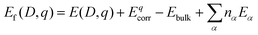(40)
and the stability of a defective structure in equilibrium with its environment is given by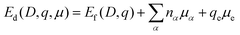(41)
where E(D,q) is the total energy of a supercell having defect D and extra charge q, Ebulk is the energy of the host material without any defect, nα is the number of atoms removed from cell and added to the reservoir, Eα is the total energy of their standard state, Eqcorr is the correction term for the interactions of the image charges,93qe is the number of electrons transferred to the reservoir and μe their chemical potential with respect to valence band maxima (VBM). The chemical potential μα is controlled by the experimental growth conditions. Bera et al.91 used this formalism to find out the appropriate dopant for SnS among monovalent cations (Li, Na, K, Cu, Ag, Au, Tl, Hg) and trivalent anions (N, P, As, Sb, Bi). In the case of SnX (X = S, Se, O and Te), the chemical potential limits are given by
 μSn ≤ 0 (42)
 μX ≤ 0 (43)
 μSn + 2μX ≤ 3ΔHf(SnX2) (44)

For maintaining stability in the crystal,

 μSn + μX = 2ΔHf(SnX) (45)

The crystal structure after Ag doping and the relevant chemical potential limits for doping SnS are shown in Fig. 13a and b respectively. The calculated defect formation energy as a function of μe for all these defects in Sn-rich and S-rich limits is shown in Fig. 14. It was found that not only in the S-rich limit the unfavourable VSn destabilizes, but also the AgSn defect stabilizes. In ref. 91 defect formation energy calculation for SnSe is discussed and in ref. 38 stable defect formation in SnO is discussed.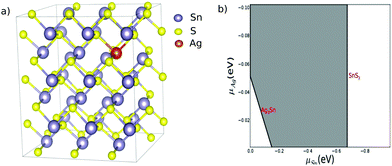Fig. 13 (a) The crystal structure of SnS showing Ag substituted on the Sn site in the SnS lattice. (b) Calculated chemical potential limits for Ag doped SnS. The shaded area is for the allowed equilibrium growth conditions. Reprint from ref. 91.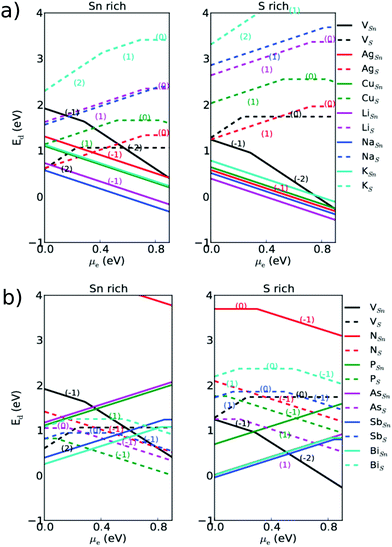Fig. 14 Defect formation energies for Sn-rich and S-rich limits in SnS as a function of the electron chemical potential. The slope of the lines corresponds to the charge of defects. The solid lines and dashed lines correspond to defects at the Sn and S position respectively. Reprint from ref. 91.

The equilibrium defect concentration can be determined from the calculated formation energies as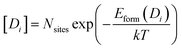(46)
where Nsites is the substitutional site concentration in the crystal and Eform is the formation energy. This equation can be solved by self-consistently imposing charge neutrality,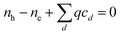(47)
where nh and ne are the number of holes and electrons given by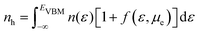(48)
and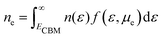(49)

In the above equations, ECBM is the energy of the conduction band minimum, n(ε) is the electronic density of states of the defect free crystal and f(ε,μe) is the Fermi distribution. Fig. 15 shows the calculated carrier concentration based on defect formation energies in SnS and SnSe, which indicates that intrinsic carrier concentration in the low-temperature phase of SnSe (∼1018 cm−3) is two orders of magnitude higher than in SnS (∼1016 cm−3) and carrier concentration in the low temperature phase (Pnma structure) of SnSe can still be further optimized by silver doping.91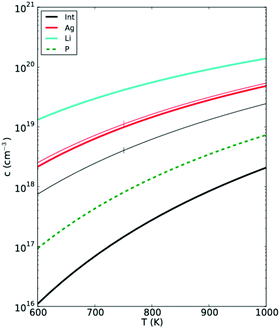Fig. 15 Carrier concentration due to defects in SnS as a function of fabrication temperature. All lines correspond to the S-rich limit except for phosphor doping, which is in the Sn-rich limit. Also shown in thin lines are the calculated carrier concentrations in the low temperature phase of SnSe. Reprint from ref. 91.

Distortion of the electronic density of states (DOS) is also a potent mechanism to increase the thermopower of thermoelectric semiconductors. One complementary band-structure engineering approach that promises to enhance the thermoelectric power is the use of ‘resonant impurities’ as dopants. The review by Nemov94 showed that resonant impurities are a concept that was introduced in solid state physics for metals first, and they also exist in many semiconductors. L. A. Falk’ovskii pointed out on a theoretical basis that resonant levels (RL) are likely to exist in very narrow-gap semiconductors, particularly those with a strongly non-parabolic dispersion relation.95

The narrower the gap, the higher the probability that an impurity level will coincide with a band. RLs involve a coupling between electrons of the impurity and electrons in the conduction or the valence band of the host solid. The impurity atoms have multiple electron energy levels corresponding to either a bound state or an extended state. Resonant donor impurities would have electronic energy levels for which ED (equivalent to the thermal excitation energy of dopants in conventional semiconductors) is negative or positive (depends on donor or acceptor type), with the impurity level falling inside the conduction band or valence band and coinciding with energies of extended states.96 This state is called a resonant state. As this state has now the same energy as an extended state, the two will resonate to build up two extended states of slightly different energies; these in turn will have the same energies as other extended states with which they will resonate in turn, and so on. Consequently, the resonant state develops a certain width Γ. Both ED and Γ are essential design parameters in optimizing ZT. Mahan and Sofo showed that, the narrower the Γ, the higher the ZT.97 The resonant level enhances the thermoelectric properties due to its excess density of states at their corresponding energy levels and the resonant state diffuses conduction electrons in a way that is extremely sensitive to their energy known as resonant scattering. The chalcogens S, Se, and Te are mentioned as potential resonant donors in heavy compounds like InAs and InSb.97 A significantly enhanced Seebeck coefficient of 116 μV K−1 at 300 K was observed by codoping of Mn and In in SnTe due to a hump in DOS due to resonance levels.98 Al doping in PbSe created resonant states in the conduction band resulting in an increase of the local density of states near the Fermi level causing the Seebeck coefficient to be about 40% higher than that of the Cl-doped PbSe sample without resonant states.99 These studies suggest that resonant impurity levels can be used to maximize the thermoelectric figure of merit, by maximizing the thermoelectric power at a given carrier concentration.

Doping in SnX (X = S, Se, O and Te) is discussed here to understand the importance of doping and defect engineering. Na doped SnS also has very good thermoelectric properties and has ZT = 0.65 due to its increased carrier concentration (1019 cm−3).100 Further, a very high ZT = 1.6 was reported for substituting S with Se by Wenke et al.23 A first principles study of Bi doped SnS showed that the ZT value increased from 0.16 to 0.36 at 1.56% Bi concentration.101 The study of In (5% and 15%) doped SnS samples and Sb (5% and 15%) doped SnS samples showed an increase in electrical conductivity and Seebeck coefficient with temperature indicating p-type semiconducting behaviour of all samples.102

A high figure of merit (ZT) = 1.1 at 773 K was reported in n-type Sb doped SnSe microplates due to high carrier concentration (3.94 × 1019 cm−3)103 and ZT = 2.2 was obtained at 733 K for Bi doped n-type SnSe crystals having a carrier density of 2.1 × 1019 cm−3.104 An experimental study also showed that for 2 wt% Cu doped SnSe, a ZT value of 0.7 ± 0.02 can be obtained at 773 K.105

It was found by Tan et al. that Bi and In co-doping in SnTe can enhance the Seebeck coefficient, resulting in a significant increase in the ZT value to 1.26 at 900 K.106 Mn, Bi and Sb co-doped SnTe has low lattice thermal conductivity and ZT ∼ 1 at 773 K107 indicating it to be a good thermoelectric material. All of the above mentioned studies suggest that SnS, SnSe, SnO and SnTe are good thermoelectric materials and can be used to design and optimize the advanced thermoelectric devices.

Our theoretical calculations based on relaxation time approximation and the rigid band model show that extrinsic doping largely affects the ion-impurity scattering rate. In Sn-based chalcogenides, electrical properties can be improved via extrinsic doping. Theoretical studies show ∼102 times improvement for p-type doping and ∼10 times improvement for n-type doping in SnSe0.70S0.30.30

### 6.2 Alloying and solid solution

Alloying is either substitution of other atoms in the host crystal structure at lattice sites (substitutional alloying) or occupation of guest atoms at interstitial sites in the host crystal structure (interstitial alloying). This has been shown diagrammatically in Fig. 16.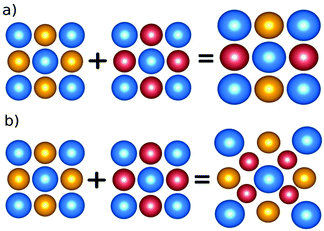Fig. 16 Different types of alloying. (a) Substitutional – the red atom occupies the lattice site of the yellow atom in the host crystal structure. (b) Interstitial – the red atom occupies the interstitial site of the host structure.

Substitutional alloying has a remarkable effect on the thermoelectric behaviour of the material as it produces different kinds of defect atoms on lattice sites changing volume and hence thermoelectric properties.108 Formation of an alloy is conditioned to the structure of components forming the alloys. The crystal structure of the forming materials should be approximately similar. This is generally achieved by choosing materials with iso-electronic elements as they tend to make a similar kind of structure.

Among chalcogenides of tin (Sn), SnS has orthorhombic structure with space group Pnma and is being continuously investigated as a thermoelectric material.109 SnSe also has Pnma structure.110 Wang et al.111 showed that in the case of undoped SnS, which possesses low carrier concentration and inferior TE properties, its thermoelectric performance can be improved by Se alloying. They showed that narrowing of the band gap and flattening of the valence band shape contribute to excellent electrical transport properties, resulting in a maximum power factor of ∼6.0 mW cm−1 K−2 and a record high ZT of 0.70 at 873 K. In another report112 the thermal conductivity of SnSe was greatly reduced upon Te substitution (SnSe1−xTex) due to alloy scattering of phonons as well explained by the Debye model. Due to the increased carrier concentration by Na-doping in this alloy compound, the thermoelectric figure of merit (ZT) was enhanced in the whole temperature range with a maximum value of 0.72 obtained at a relatively low temperature (773 K) for Sn0.99Na0.01Se0.84Te0.16.

In another Sn based non-layered chalcogenide, SnTe alloying was found to be very important for optimizing TE properties. Zhang et al.14 reported that both the power factor and thermal conductivity of SnTe can be simultaneously improved by introducing its analogues (PbTe, PbSe, PbS, SnSe, and SnS) into the SnTe matrix. They found that the power factor of SnTe is greatly enhanced as alloying with its analogues modifies its band structure. They showed that Seebeck coefficients at room temperature show an increasing trend with increasing PbTe fractions. The Seebeck coefficient increases from ∼ 170 μV K−1 for Sn0.97Bi0.03Te to ∼200 μV K−1 for Sn0.97Bi0.03Te with 3 wt% PbTe at 900 K. This increase is the result of convergence of the two valence bands, the light valence band (L) and the heavy valence band (Σ), which facilitates charge carrier injection and the participation of the heavy hole band in the carrier transport leads to increment in power factor values and hence higher figure of merit. An increase in electrical conductivity is also seen for SnO when it is alloyed with SnSe and SnS as seen in Fig. 7b.

Alloying also plays a major role in reducing the lattice thermal conductivity. It affects a particular range of phonons and scatters them selectively, thus enhancing the thermoelectric figure of merit. In the literature, the thermal conductivity of binary compounds SnS and SnSe is reduced when alloy SnSe1−xSx is formed. Gupta et al.30 show that the thermal conductivity of SnSe0.70S0.30 is 1.3 times lower than that of SnSe and ∼2 times lower than that of SnS. This reduction in thermal conductivity for the alloy case is mainly due to atomic mass difference, which increases scattering and hence impedes heat transport by short wavelength phonons. The lattice thermal conductivity also decreased in SnO alloys SnO0.70Se0.30 and SnO0.70S0.30 giving higher ZT compared to pure SnO as seen in Fig. 17. ZT for SnO0.70S0.30 is higher at 300 K but is the same at 800 K. SnO and SnSe alloying enhances the figure of merit twice from 0.0022 to 0.0044 at 800 K.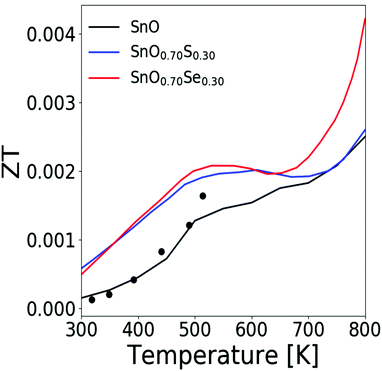Fig. 17 Variation of figure of merit with temperature for SnO, SnO0.70Se0.30 and SnO0.70S0.30 with experimental values for pure SnO.38

Thus, alloying can enhance the thermoelectric properties of materials. It leads to modification of electronic band structure by shifting of light and heavy valence bands leading to band convergence which is discussed in the next subsection.

### 6.3 Band convergence

Band convergence is tuning of periodical band structure leading to the convergence of bands, for example shifting of the heavy valence band towards the light valence band as a result of heavy doping or alloying. It requires a relatively high doping level to influence the k-space band dispersion. Out of all tin chalcogenides, SnTe which has rarely been considered to be an efficient thermoelectric material due inability to control its very high carrier concentration shows a major scope for thermoelectric properties improvement by band convergence. The schematic diagram is shown in Fig. 18 where the effect of Mn doping on pure SnTe reduces the energy gap (ΔELΣ) between light-hole and heavy-hole valence bands causing band convergence.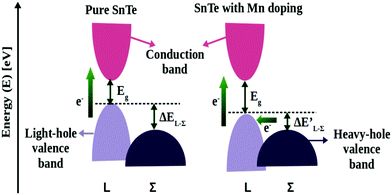Fig. 18 Schematic diagram showing band convergence in SnTe.

Band convergence can significantly enhance the Seebeck coefficient without detrimental effects on the electrical conductivity in SnTe.14 Acharya et al.28 also showed that with heavy atomic mass and strong spin–orbit coupling, even the mild doping of Yb (∼5%) is enough to create a degeneracy via band-convergence which enhances the density of states near the Fermi level and improves the overall thermoelectric response and is equivalent to 9% of Mg doping. The supercell approach is used for band convergence study in SnTe28 and PbTe.113 There is no report on band convergence for SnS, SnSe and SnO to date.

### 6.4 Nanostructuring

Though charge transport and mass transport at nanoscales have been studied extensively in microelectronics and chemical and biological sensors,114,115 nanoscale heat transport has received little attention. A detailed study of heat transport at the nanoscale is critical in improving the performance of low-dimensional devices.116 Theoretical studies of transport in lower dimensions such as nanotubes, nanowires and thin films are continuously being done to see the thermoelectric behaviour at such nanoscales.

In 1990, Hicks, Dresselhaus and Harman suggested117,118 that the figure of merit could be improved if electrons were confined in two dimensions using quantum well superlattices, where superlattices are multilayers of thin films of the order of several nanometers in thickness. Hicks and Dresselhaus later extended their work to include one-dimensional conductors such as nanowires.119 The primary reason for the enhancement in the figure of merit in low-dimensional structures is due to an increase in the electronic density of states per unit volume, which leads to an improved thermopower. Boltzmann transport equations are also applied at lower dimensions and their applicability is justified by considering carrier-boundary scattering.

Liu et al.120 recently reported SnSe/SnS hetero-nanosheet tuning by the epitaxial growth of SnSe on the few layers of SnS nanosheets. The heterojunction nano-interface optimizes the carrier/phonon transport behavior by the energy filtering effect leading to an increase in power factor from 2.2 μW cm−1 K−2 to 3.21 μW cm−1 K−2 at 773 K compared to pristine SnSe and a significant reduction in thermal conductivity from 0.65 W m−1 K−1 to 0.48 W m−1 K−1 at 773 K. They reported a maximum ZT of 0.5 at 773 K in the SnSe/SnS hetero-nanosheets, which is 89% higher than that of pristine SnSe. Hence, their approach proved to be a promising strategy to design high performance thermoelectric materials.

A recent report121 show the development of a new solution synthesizing method (in situ magnetic field-assisted hydrothermal synthesis) for achieving new nanostructured SnSe integrated with Se quantum dots. This method leads to reduction in critical nucleation energy and enhancement of the nucleation during the hydrothermal synthesis process as a high magnetic field is applied, leading to the presence of the homogeneous distribution of Se quantum dots and smaller nanograins. SnSe nanostructures and Se quantum dots (Se quantum dot/Sn0.99Pb0.01Se nanocomposite) enhance the density of states and cause the energy filtering effect which contribute towards a significant enhancement in the Seebeck coefficient and power factor which further leads to a high figure of merit (ZT) of ∼2.0 at 873 K.

Xu et al.122 adopted a strategy of interface engineering to design oriented nanopillar structure in SnTe films via the one-step thermal evaporation method. The analysis of the electrical and thermal properties illustrates that the SnTe film with highly oriented growth shows enhanced thermoelectric performance. The excellent properties are mainly attributed to the special nanopillar structure, which may facilitate electrons' transport while significantly scattering the phonons. A maximum power factor of 19.8 μW cm−1 K−2 and a thermal conductivity of 3.54 W m−1 K−1 are obtained making the work a promising approach to enhance the thermoelectric performance of SnTe films.

Considering diffusive type surface scattering no reduction in the thermal conductivity of layered tin based chalcogenides has been observed as seen in Fig. 19. Gupta et al.30 showed that a reduction of diameter to 100 nm changes the thermal conductivity of SnSe0.70S0.30 by only ∼10%, therefore not affecting the figure of merit of the materials. SnO0.70S0.30 and SnO0.70S0.30 also show the same behaviour on nanostructuring.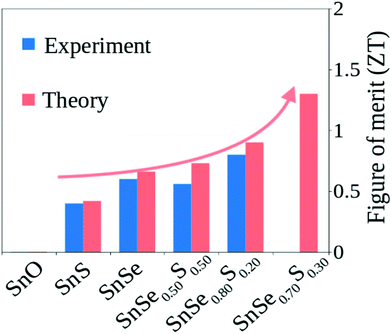Fig. 19 Bar plot showing a comparison of theoretically calculated figure of merit30 and experimental values for tin-based chalcogenides.30,38,54,55,125

As the electron generally has a smaller wavelength compared to the phonon, nanostructuring will not affect electron scattering much in Sn-based chalcogenides. Electron and phonon dispersions can also change in nanostructure systems but full ab initio calculations based on DFT will be very expensive to study the nanostructure electron and phonon dispersions. The tight binding model can be suitable for this type of calculation. So far there is no report on the electronic and phonon band structure of nanowires or nanoparticles based on Sn-based chalcogenides. Recently a few studies have reported thermoelectric properties of monolayered Sn based chalcogenide compounds.123,124

## 7 Best ZT

Fig. 19 shows the theoretically predicted figure of merit of tin-based chalcogenide materials and their alloys along with experimental values.

The theoretical model discussed in this perspective gives results well in agreement with experimental values.38,54,55,125 SnO has the lowest ZT among all other chalcogenides. The figure of merit improves on alloying with the highest value of 1.3 obtained theoretically for SnSe0.70S0.30 which is not reported yet experimentally. From theoretical calculations, it is observed that SnSe0.70S0.30 will be the best Sn-based thermoelectric material.

When the device efficiency is calculated from eqn (1), large enhancement is also observed in SnSe0.70S0.30. The variation of η/η0 with temperature for ideal ZT is shown in Fig. 20. The different lines in the graph are calculated by taking Z as constant for the entire temperature range. The scatterers represent the experimental and theoretical data for layered tin-based chalcogenides and their alloys.30,38,54,55,125η/η0 for these points was calculated by taking average of ZT values at TC = 300 K and TH = 800 K. It is seen that the alloy SnSe0.70S0.30 has the maximum efficiency of 17.20% with respect to Carnot efficiency at 800 K.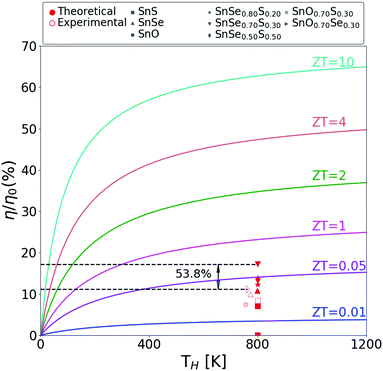Fig. 20 Variation of η/η0 with temperature for tin-based chalcogenides with theoretically calculated and experimental values.30,38,54,55,125

This is a 53.8% enhancement in the efficiency value from the experimentally reported case of SnSe0.50S0.50. From this plot it is seen that thermoelectric device efficiency with respect to Carnot efficiency can be improved in the medium temperature range by alloying and proper extrinsic doping.

## 8 Conclusions and outlook

To conclude, we have discussed the theoretical model for calculating thermoelectric properties in general with the main emphasis on tin-based chalcogenides. The electron transport is modelled by including various scattering mechanisms like electron–phonon scattering, ion-impurity scattering and alloy scattering. Though the scattering rate is approximated considering a simple model, still it gives results well in agreement (more than 90%) with the experimental values. These scattering calculations are also faster compared to the full first-principles scattering calculation technique.126 The phonon transport, on the other hand, is modelled by taking Umklapp and Normal scattering processes into account. The latter plays an important role in the case of layered compounds where inclusion of just Umklapp scattering overestimates the lattice thermal conductivity. Alloy scattering dominates both these scattering processes and reduces the lattice thermal conductivity. The effect of alloying, intrinsic and extrinsic defects and nanostructuring for improving the figure of merit of tin-based chalcogenides by proper optimization of properties has also been discussed. While doping with silver enhances the electrical conductivity of SnO, SnS and SnSe by two to three orders of magnitude, alloying helps in reducing the lattice thermal conductivity. Though a two-times increase in ZT from pure SnO is observed for SnO alloying with SnSe (SnO0.70Se0.30), the ZT is still low due to SnO's high thermal conductivity and low carrier concentration. The best ZT value of 1.3 is achieved by SnS and SnSe alloying (SnSe0.70S0.30) at 800 K which corresponds to 17.20% TE device efficiency with respect to Carnot efficiency. The efficiency of these chalcogenides can be further improved by tuning carrier concentration in the alloy. This work will give insight into details of the electron and phonon transport model and various ways of optimizing figure of merit. This model will be useful for the fast prediction of optimum thermoelectric properties and will encourage further investigation of thermoelectric properties of tin-based or other transition metal chalcogenides.

## Code availability

All codes are available from the corresponding author on request.

## Conflicts of interest

There are no conflicts to declare.

## Acknowledgements

The authors acknowledge financial support from SERB, DST, India (project No. SERB-EMR/2016/003584 and SERB-EEQ/2016/000499). NK acknowledge PhD fellowship from CSIR, India (grant no. 09/1129(0017)/2019-EMR-I).

## Notes and references

1. A. Grubler, C. Wilson, N. Bento, B. Boza-Kiss, V. Krey, D. L. McCollum, N. D. Rao, K. Riahi, J. Rogelj, S. D. Stercke, J. Cullen, S. Frank, O. Fricko, F. Guo, M. Gidden, P. Havlík, D. Huppmann, G. Kiesewetter, P. Rafaj, W. Schoepp and H. Valin, Nat. Energy, 2018, 3, 515–527 CrossRef.
2. K. Biswas, J. He, I. D. Blum, C. I. Wu, T. P. Hogan, D. N. Seidman, V. P. Dravid and M. G. Kanatzidis, Nature, 2012, 489, 414 CrossRef CAS PubMed.
3. C. J. Vineis, A. Shakouri, A. Majumdar and M. G. Kanatzidis, Adv. Mater., 2010, 22, 3970–3980 CrossRef CAS PubMed.
4. D. Wang, W. Shi, J. Chen, J. Xi and Z. Shuai, Phys. Chem. Chem. Phys., 2012, 14, 16505–16520 RSC.
5. J. Yang and T. Caillat, MRS Bull., 2006, 31, 224–229 CrossRef CAS.
6. H. S. Kim, W. Liu, G. Chen, C.-W. Chu and Z. Ren, Proc. Natl. Acad. Sci. U. S. A., 2015, 112, 8205–8210 CrossRef CAS PubMed.
7. P. Kaur and C. Bera, Phys. Chem. Chem. Phys., 2017, 19, 24928 RSC.
8. G. A. Slack and M. A. Hussain, J. Appl. Phys., 1991, 70, 2694–2718 CrossRef CAS.
9. J. R. Szczech, J. M. Higgins and S. Jin, J. Mater. Chem., 2011, 21, 4037–4055 RSC.
10. G. Snyder and E. Toberer, Nat. Mater., 2008, 7, 105–114 CrossRef CAS PubMed.
11. L.-D. Zhao, G. Tan, S. Hao, J. He, Y. Pei, H. Chi, H. Wang, S. Gong, H. Xu and V. P. Dravid, et al. , Science, 2016, 351, 141–144 CrossRef CAS PubMed.
12. J. Zhang, X. Liu, Y. Wen, L. Shi, R. Chen, H. Liu and B. Shan, ACS Appl. Mater. Interfaces, 2017, 9, 2509–2515 CrossRef CAS PubMed.
13. Y. Cheng, J. Yang, Q. Jiang, D. He, J. He, Y. Luo, D. Zhang, Z. Zhou, Y. Ren and J. Xin, J. Mater. Chem. A, 2017, 5, 5163–5170 RSC.
14. X. Zhang, D. Wang, H. Wu, M. Yin, Y. Pei, S. Gong, L. Huang, S. J. Pennycook, J. He and L.-D. Zhao, Energy Environ. Sci., 2017, 10, 2420–2431 RSC.
15. L.-D. Zhao, S.-H. Lo, Y. Zhang, H. Sun, G. Tan, C. Uher, C. Wolverton, V. P. Dravid and M. G. Kanatzidis, Nature, 2014, 508, 373–377 CrossRef CAS PubMed.
16. J.-H. Yang, Q. Yuan, H. Deng, S.-H. Wei and B. I. Yakobson, J. Phys. Chem. C, 2017, 121, 123–128 CrossRef CAS.
17. X.-L. Zhu, P.-F. Liu, J. Zhang, P. Zhang, W.-X. Zhou, G. Xie and B.-T. Wang, Nanoscale, 2019, 11, 19923–19932 RSC.
18. F. Q. Wang, Y. Guo, Q. Wang, Y. Kawazoe and P. Jena, Chem. Mater., 2017, 29, 9300–9307 CrossRef CAS.
19. H.-J. Wu, P.-C. Wei, H.-Y. Su, K.-K. Wang, W.-T. Yen, I.-L. Jen and J. He, ACS Appl. Energy Mater., 2019, 2, 7564–7571 CrossRef CAS.
20. X. Chen, J. Zhou, J. B. Goodenough and L. Shi, J. Mater. Chem. C, 2015, 3, 10500–10508 RSC.
21. J. M. Ma, S. M. Clarke, W. G. Zeier, T. Vo, P. Von Allmen, G. J. Snyder, R. B. Kaner, J.-P. Fleurial and S. K. Bux, J. Mater. Chem. C, 2015, 3, 10459–10466 RSC.
22. S. Heimann, S. Schulz, J. Schaumann, A. Mudring, J. Stötzel, F. Maculewicz and G. Schierning, J. Mater. Chem. C, 2015, 3, 10375–10380 RSC.
23. W. He, D. Wang, H. Wu, Y. Xiao, Y. Zhang, D. He, Y. Feng, Y.-J. Hao, J.-F. Dong and R. Chetty, et al. , Science, 2019, 365, 1418–1424 CrossRef CAS PubMed.
24. S. Li, J. Yang, J. Xin, Q. Jiang, Z. Zhou, H. Hu, B. Sun, A. Basit and X. Li, ACS Appl. Energy Mater., 2019, 2, 1997–2003 CrossRef CAS.
25. S. Hao, F. Shi, V. P. Dravid, M. G. Kanatzidis and C. Wolverton, Chem. Mater., 2016, 28, 3218–3226 CrossRef CAS.
26. C. Liu, Z. Huang, D. Wang, X. Wang, L. Miao, X. Wang, S. Wu, N. Toyama, T. Asaka and J. Chen, et al. , J. Mater. Chem. A, 2019, 7, 9761–9772 RSC.
27. K. Zhao, K. Liu, Z. Yue, Y. Wang, Q. Song, J. Li, M. Guan, Q. Xu, P. Qiu, H. Zhu, L. Chen and X. Shi, Adv. Mater., 2019, 31, 1903480 CrossRef CAS PubMed.
28. S. Acharya, D. Dey, T. Maitra, A. Soni and A. Taraphder, Appl. Phys. Lett., 2018, 113, 193904 CrossRef.
29. G. Kresse and D. Joubert, Phys. Rev. B: Condens. Matter Mater. Phys., 1999, 59, 1758 CrossRef CAS.
30. R. Gupta, B. Kaur, J. Carrete and C. Bera, J. Appl. Phys., 2019, 126, 225105 CrossRef.
31. T. Chattopadhyay, J. Pannetier and H. V. Schnering, J. Phys. Chem. Solids, 1986, 47, 879–885 CrossRef CAS.
32. H. Wiedemeier, H. Georg and G. Schnering, Z. Kristallogr., 1978, 3, 295 CrossRef.
33. W. Albers, C. Haas, H. J. Vink and J. D. Wasscher, J. Appl. Phys., 1961, 32, 2220 CrossRef CAS.
34. M. Parenteau and C. Carlone, Phys. Rev. B: Condens. Matter Mater. Phys., 1990, 41, 5227–5234 CrossRef CAS PubMed.
35. J. P. Perdew, K. Burke and M. Ernzerhof, Phys. Rev. Lett., 1996, 77, 3865 CrossRef CAS PubMed.
36. P. E. Blöchl, Phys. Rev. B: Condens. Matter Mater. Phys., 1994, 50, 17953 CrossRef PubMed.
37. M. S. Moreno and R. C. Mercader, Phys. Rev. B: Condens. Matter Mater. Phys., 1994, 50, 9875–9881 CrossRef CAS PubMed.
38. S. A. Miller, P. Gorai, U. Aydemir, T. O. Mason, V. Stevanović, E. S. Toberer and G. J. Snyder, J. Mater. Chem. C, 2017, 5, 8854–8861 RSC.
39. B. Gelmont, K. Kim and M. Shur, J. Appl. Phys., 1993, 74, 1818–1821 CrossRef CAS.
40. J. M. Ziman, Electrons and phonons: the theory of transport phenomena in solids, Clarendon Press, Oxford, 1960 Search PubMed.
41. B. R. Nag, Electron transport in compound semiconductors, Springer-Vlg, 1980 Search PubMed.
42. M.-S. Jeng, R. Yang and G. Chen, ICT 2005. 24th International Conference on Thermoelectrics, 2005.
43. C. Bera, J. Appl. Phys., 2012, 112, 074323 CrossRef.
44. J. M. Ziman, Can. J. Phys., 1956, 34, 1256–1273 CrossRef.
45. A. Casian, I. Sur, H. Scherrer and Z. Dashevsky, Phys. Rev. B: Condens. Matter Mater. Phys., 2000, 61, 15965–15974 CrossRef CAS.
46. D. L. Rode, Phys. Rev. B: Condens. Matter Mater. Phys., 1970, 2, 1012–1024 CrossRef.
47. K. Rektorys, Survey of Applicable Mathematics, Springer, Netherlands, 1994 Search PubMed.
48. D. L. Rode and S. Knight, Phys. Rev. B: Condens. Matter Mater. Phys., 1971, 3, 2534–2541 CrossRef.
49. D. L. Rode, Phys. Rev. B: Condens. Matter Mater. Phys., 1970, 2, 4036–4044 CrossRef.
50. J. Ziman, Electrons and Phonons, the Theory of Transport Phenomena in Solids, 1979 Search PubMed.
51. N. M. Ravindra, B. Jariwala, A. Bañobre and A. Maske, Thermoelectrics, Springer International Publishing, 2019 Search PubMed.
52. C. Bera, M. Soulier, C. Navone, G. Roux, J. Simon, S. Volz and N. Mingo, J. Appl. Phys., 2010, 108, 124306 CrossRef.
53. R. L. González-Romero, A. Antonelli and J. J. Meléndez, Phys. Chem. Chem. Phys., 2017, 19, 12804–12815 RSC.
54. Q. Tan and J. F. Li, J. Electron. Mater., 2014, 43, 2435 CrossRef CAS.
55. C. L. Chen, H. Wang, Y. Y. Chen, T. Day and G. J. Snyder, J. Mater. Chem. A, 2014, 2, 11171 RSC.
56. G. K. H. Madsen, J. Carrete and M. J. Verstraete, Comput. Phys. Commun., 2018, 231, 140 CrossRef CAS.
57. P. Graziosi, C. Kumarasinghe and N. Neophytou, J. Appl. Phys., 2019, 126, 155701 CrossRef.
58. J. Appel, Phys. Rev., 1961, 122, 1760–1772 CrossRef CAS.
59. H. Meijer and D. Polder, Physica, 1953, 19, 255–264 CrossRef CAS.
60. J. D. Zook, Phys. Rev., 1964, 136, A869–A878 CrossRef.
61. J. Bardeen and W. Shockley, Phys. Rev., 1950, 80, 72 CrossRef CAS.
62. A. Togo, F. Oba and I. Tanaka, Phys. Rev. B: Condens. Matter Mater. Phys., 2008, 78, 134106 CrossRef.
63. H. Brooks and C. Herring, Phys. Rev., 1951, 83, 879 CAS.
64. J. W. Harrison and J. R. Hauser, Phys. Rev. B: Condens. Matter Mater. Phys., 1976, 13, 5347 CrossRef CAS.
65. P. A. Flinn, Phys. Rev., 1956, 104, 350 CrossRef CAS.
66. W. A. Harrison, Phys. Rev., 1956, 104, 1281–1290 CrossRef CAS.
67. J. Cao, J. D. Querales-Flores, A. R. Murphy, S. Fahy and I. Savić, Phys. Rev. B, 2018, 98, 205202 CrossRef.
68. G. Samsonidze and B. Kozinsky, Adv. Energy Mater., 2018, 8, 1800246 CrossRef.
69. Z. Wang, S. Wang, S. Obukhov, N. Vast, J. Sjakste, V. Tyuterev and N. Mingo, Phys. Rev. B, 2011, 83, 205208 CrossRef.
70. B. Qiu, Z. Tian, A. Vallabhaneni, B. Liao, J. M. Mendoza, O. D. Restrepo, X. Ruan and G. Chen, EPL, 2015, 109, 57006 CrossRef.
71. J.-J. Zhou, J. Park, I.-T. Lu, I. Maliyov, X. Tong and M. Bernardi, Perturbo: a software package for ab initio electron–phonon interactions, charge transport and ultrafast dynamics, 2020 Search PubMed.
72. P. Kaur, G. K. H. Madsen and C. Bera, MRS Commun., 2019, 9, 370 CrossRef CAS.
73. G. K. H. Madsen, A. Katre and C. Bera, Phys. Status Solidi A, 2016, 213, 802 CrossRef CAS.
74. C. Toher, J. J. Plata, O. Levy, M. de Jong, M. Asta, M. B. Nardelli and S. Curtarolo, Phys. Rev. B, 2014, 90, 174107 CrossRef.
75. E. S. Toberer, A. Zevalkink and G. J. Snyder, J. Mater. Chem., 2011, 21, 15843 RSC.
76. S. A. Miller, P. Gorai, B. R. Ortiz, A. Goyal, D. Gao, S. A. Barnett, T. O. Mason, G. J. Snyder, Q. Lv, V. Stevanović and E. S. Toberer, Chem. Mater., 2017, 29, 2494–2501 CrossRef CAS.
77. D. T. Morelli, J. P. Heremans and G. A. Slack, Phys. Rev. B: Condens. Matter Mater. Phys., 2002, 66, 195304 CrossRef.
78. P. G. Klemens, Proc. Phys. Soc., London, Sect. A, 1955, 68, 1113–1128 CrossRef.
79. C. A. Ratsifaritana and P. G. Klemens, Int. J. Thermophys., 1987, 8, 737–750 CrossRef CAS.
80. L. Bjerg, B. B. Iversen and G. K. H. Madsen, Phys. Rev. B: Condens. Matter Mater. Phys., 2014, 89, 024304 CrossRef.
81. G. Tan, L.-D. Zhao, F. Shi, J. W. Doak, S.-H. Lo, H. Sun, C. Wolverton, V. P. Dravid, C. Uher and M. G. Kanatzidis, J. Am. Chem. Soc., 2014, 136, 7006–7017 CrossRef CAS PubMed.
82. W. Li, Z. Chen, S. Lin, Y. Chang, B. Ge, Y. Chen and Y. Pei, J. Materiomics, 2015, 1, 307–315 CrossRef.
83. J. Carrete, B. Vermeersch, A. Katre, A. van Roekeghem, T. Wang, G. K. Madsen and N. Mingo, Comput. Phys. Commun., 2017, 220, 351–362 CrossRef CAS.
84. W. Li, J. Carrete, N. A. Katcho and N. Mingo, Comput. Phys. Commun., 2014, 185, 1747–1758 CrossRef CAS.
85. B. Dongre, J. Carrete, S. Wen, J. Ma, W. Li, N. Mingo and G. K. H. Madsen, J. Mater. Chem. A, 2020, 8, 1273–1278 RSC.
86. C. G. Van de Walle, D. Laks, G. Neumark and S. Pantelides, Phys. Rev. B: Condens. Matter Mater. Phys., 1993, 47, 9425 CrossRef CAS.
87. S. Zhang and J. E. Northrup, Phys. Rev. Lett., 1991, 67, 2339 CrossRef CAS PubMed.
88. R. Chmielowski, S. Bhattacharya, W. Xie, D. Pere, S. Jacob, R. Stern, K. Moriya, A. Weidenkaff, G. Madsen and G. Dennler, J. Mater. Chem. C, 2016, 4, 3094–3100 RSC.
89. P. Hohenberg and W. Kohn, Phys. Rev., 1964, 136, B864 CrossRef.
90. D. Hamann, M. Schlüter and C. Chiang, Phys. Rev. Lett., 1979, 43, 1494 CrossRef CAS.
91. C. Bera, S. Jacob, I. Opahle, N. H. Gunda, R. Chmielowski, G. Dennler and G. K. Madsen, Phys. Chem. Chem. Phys., 2014, 16, 19894–19899 RSC.
92. D. Laks, C. Van de Walle, G. Neumark, P. Blöchl and S. Pantelides, Phys. Rev. B: Condens. Matter Mater. Phys., 1992, 45, 10965 CrossRef CAS PubMed.
93. H.-P. Komsa, T. T. Rantala and A. Pasquarello, Phys. Rev. B: Condens. Matter Mater. Phys., 2012, 86, 045112 CrossRef.
94. S. Nemov and Y. I. Ravich, Phys.-Usp., 1998, 41, 735 CrossRef.
95. L. Fal'kovskil, Zh. Eksp. Teor. Fiz., 1975, 68, 1529–1538 Search PubMed.
96. J. P. Heremans, B. Wiendlocha and A. M. Chamoire, Energy Environ. Sci., 2012, 5, 5510–5530 RSC.
97. G. Mahan and J. O. Sofo, Proc. Natl. Acad. Sci. U. S. A., 1996, 93, 7436–7439 CrossRef CAS PubMed.
98. L. Wang, X. Tan, G. Liu, J. Xu, H. Shao, B. Yu, H. Jiang, S. Yue and J. Jiang, ACS Energy Lett., 2017, 2, 1203–1207 CrossRef CAS.
99. Q. Zhang, H. Wang, W. Liu, H. Wang, B. Yu, Q. Zhang, Z. Tian, G. Ni, S. Lee and K. Esfarjani, et al. , Energy Environ. Sci., 2012, 5, 5246–5251 RSC.
100. B. Zhou, S. Li, W. Li, J. Li, X. Zhang, S. Lin, Z. Chen and Y. Pei, ACS Appl. Mater. Interfaces, 2017, 9, 34033–34041 CrossRef CAS PubMed.
101. J. Guan, Z. Zhang, M. Dou, J. Ji, Y. Song, J. Liu, Z. Li and F. Wang, J. Phys. Chem. Solids, 2020, 137, 109182 CrossRef CAS.
102. S. H. Chaki, M. D. Chaudhary and M. Deshpande, Mater. Res. Bull., 2015, 63, 173–180 CrossRef CAS.
103. X.-L. Shi, K. Zheng, W.-D. Liu, Y. Wang, Y.-Z. Yang, Z.-G. Chen and J. Zou, Adv. Energy Mater., 2018, 8, 1800775 CrossRef.
104. A. T. Duong, G. Duvjir, S. Kwon, J. Y. Song, J. K. Lee, J. E. Lee, S. Park, T. Min, J. Lee and J. Kim, et al. , Nat. Commun., 2016, 7, 1–6 Search PubMed.
105. N. K. Singh, S. Bathula, B. Gahtori, K. Tyagi, D. Haranath and A. Dhar, J. Alloys Compd., 2016, 668, 152–158 CrossRef CAS.
106. H. Tan, L. Guo, G. Wang, H. Wu, X. Shen, B. Zhang, X. Lu, G. Wang, X. Zhang and X. Zhou, ACS Appl. Mater. Interfaces, 2019, 11, 23337–23345 CrossRef CAS PubMed.
107. S. K. Kihoi, H. Kim, H. Jeong, H. Kim, J. Ryu, S. Yi and H. S. Lee, J. Alloys Compd., 2019, 806, 361–369 CrossRef CAS.
108. X. Lu, D. T. Morelli, Y. Wang, W. Lai, Y. Xia and V. Ozolins, Chem. Mater., 2016, 28, 1781–1786 CrossRef CAS.
109. Y. Zhang, P. Zhu, L. Huang, J. Xie, S. Zhang, G. Cao and X. Zhao, Adv. Funct. Mater., 2014, 25, 481–489 CrossRef.
110. L. Zhao, M. Yosef, M. Steinhart, P. Göring, H. Hofmeister, U. Gösele and S. Schlecht, Angew. Chem., Int. Ed., 2006, 45, 311–315 CrossRef CAS PubMed.
111. Z. Wang, D. Wang, Y. Qiu, J. He and L.-D. Zhao, J. Alloys Compd., 2019, 789, 485–492 CrossRef CAS.
112. T.-R. Wei, C.-F. Wu, X. Zhang, Q. Tan, L. Sun, Y. Pan and J.-F. Li, Phys. Chem. Chem. Phys., 2015, 17, 30102–30109 RSC.
113. L. D. Zhao, H. J. Wu, S. Q. Hao, C. I. Wu, X. Y. Zhou, K. Biswas, J. Q. He, T. P. Hogan, C. Uher, C. Wolverton, V. P. Dravid and M. G. Kanatzidis, Energy Environ. Sci., 2013, 6, 3346 RSC.
114. C. Jacoboni and L. Reggiani, Rev. Mod. Phys., 1983, 55, 645–705 CrossRef CAS.
115. L. Esaki, Nanostruct. Mater., 1999, 12, 1–8 CrossRef.
116. R. Prasher, J. Appl. Phys., 2006, 100, 064302 CrossRef.
117. L. D. Hicks and M. S. Dresselhaus, Phys. Rev. B: Condens. Matter Mater. Phys., 1993, 47, 12727–12731 CrossRef CAS PubMed.
118. L. D. Hicks, T. C. Harman and M. S. Dresselhaus, Appl. Phys. Lett., 1993, 63, 3230–3232 CrossRef CAS.
119. L. D. Hicks and M. S. Dresselhaus, Phys. Rev. B: Condens. Matter Mater. Phys., 1993, 47, 16631–16634 CrossRef CAS PubMed.
120. X. Liu, S. Li, T. Liu, W. Zhu, R. Wang, Y. Xiao and F. Pan, Funct. Mater. Lett., 2018, 11, 1850069 CrossRef CAS.
121. R. Xu, L. Huang, J. Zhang, D. Li, J. Liu, J. Liu, J. Fang, M. Wang and G. Tang, J. Mater. Chem. A, 2019, 7, 15757–15765 RSC.
122. S. Xu, W. Zhu, H. Zhao, L. Xu, P. Sheng, G. Zhao and Y. Deng, J. Alloys Compd., 2018, 737, 167–173 CrossRef CAS.
123. S.-D. Guo and Y.-H. Wang, J. Appl. Phys., 2017, 121, 034302 CrossRef.
124. B. Dong, Z. Wang, N. T. Hung, A. R. Oganov, T. Yang, R. Saito and Z. Zhang, Phys. Rev. Mater., 2019, 3, 013405 CrossRef CAS.
125. Y. M. Han, J. Zhao, M. Zhou, X. X. Jiang, H. Q. Leng and L. F. Li, J. Mater. Chem. A, 2015, 3, 4555 RSC.
126. S. Poncé, E. Margine, C. Verdi and F. Giustino, Comput. Phys. Commun., 2016, 209, 116–133 CrossRef.
 This journal is © the Owner Societies 2020